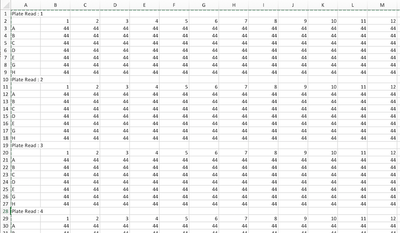Our World Statistics Day conversations have been a great reminder of how much statistics can inform our lives. Do you have an example of how statistics has made a difference in your life? Share your story with the Community!
Choose Language Hide Translation Bar
Importing Data from Plate Readers: Unfortunate Format #1: "Multiple Boxes"

I'm not sure what the most proper name for the format of data is, but I refer to it as, "multiple boxes".  In this format each reading of the plate is output into a grid. or box with the row and column label, along with the read number. Each box is separated by one empty row.

There is also an assumption that the raw data starts at A:1, that is, in Excel on the first row of the table in the first column of the table.The data formatting goal is to convert this format into the minimum number of columns, where there is one variable per column, and one observation per row.

To use the attached script, go into Excel, and select the whole page, and copy it (edit, copy). Then go in to JMP and run the script.

The script has some interesting parts, like it makes a table from the data saved to the clipboard.

All the rest of it is just basic formatting and logic to get the data into a useful shape.

rev 2 note: 2nd version of the script works better

``````Names Default To Here( 1 );
dt = New Table( "raw data" );
dt << Bring Window To Front;

dt1 = Current Data Table() << Stack(
columns(
:Column 2,
:Column 3,
:Column 4,
:Column 5,
:Column 6,
:Column 7,
:Column 8,
:Column 9,
:Column 10,
:Column 11,
:Column 12,
:Column 13
),
Source Label Column( "Label" ),
Stacked Data Column( "Data" )
);

dt1 = Current Data Table();

dt1 << New Column( "temp1", formula( If( Length( :Column 1, ) > 1, :Column 1, Lag( :temp1, 1 ) ) ) );

dt1 << New Column( "temp2", formula( If( :Column 1 != Lag( :Column 1, 1 ), 1, Lag( :temp2, 1 ) + 1 ) ) );

dt1 << New Column( "temp3", formula( If( :Column 1 == "" | Contains( :Column 1, ":" ) > 0, 1, 0 ) ) );

dt1 << New Column( "Well ID", formula( :Column 1 || Char( :temp2 ) ) );

dt1 << run formulas();

dt1:temp2 << Delete Formula;
dt1:temp3 << Delete Formula;
dt1:temp1 << set name( "Plate ID" );
:name("Well ID") << Delete Formula;
:name("Plate ID") << Delete Formula;

dt1 << Select Where( is missing (:Data) == 1 | is missing(:Column 1)==1);
dt1 << delete rows;

dt1:Column1 << set name( "Row ID" );
dt1:temp2 << set name( "Column ID" );

:Data<<set selected;
:name("Plate ID")<<set selected;
:name("Well ID")<<set selected;
dt1 << Move Selected Columns( To first );

n=ncol(dt1)-3;
dt1<< Clear Column Selection();
for (i=1, i<=n, i++,
column(dt1,4)<<set selected;
dt1 << Delete Columns();
);``````

Article Labels

There are no labels assigned to this post.

Article Tags